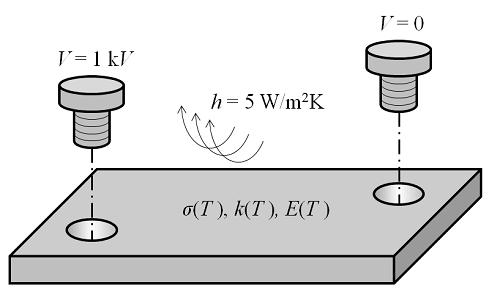# 如何处理多物理场问题

2013年 12月 16日

### 一个简单的稳态多物理场问题#### 涉及的方程

\nabla \cdot[- \sigma(T) \nabla V] = 0

\mathbf{f}_V = \mathbf{K}_V(\mathbf{u}_T)\mathbf{u}_V-\mathbf{b}_V

Q=\sigma(T)\bf{E \cdot E}

\nabla \cdot [ – k(T) \nabla T ] = Q(V)

\mathbf{f}_T = \mathbf{K}_T(\mathbf{u}_T)\mathbf{u}_T-\mathbf{b}_T(\mathbf{u}_V)

\nabla \cdot [\mathbf{C}:(\epsilon-\epsilon_{\Delta T})] = \mathbf{0}

\mathbf{f}_D = \mathbf{K}_D(\mathbf{u}_T)\mathbf{u}_D-\mathbf{b}_D(\mathbf{u}_T)

$\mathbf{f} = \left\{ \begin{array}{c} \mathbf{f}_V \\ \mathbf{f}_T \\ \mathbf{f}_d \end{array} \right\} = \left[ \begin{array}{ccc} \mathbf{K}_V(\mathbf{u}_T) %26 \mathbf{0} %26 \mathbf{0} \\ \mathbf{0} %26 \mathbf{K}_T(\mathbf{u}_T) %26 \mathbf{0} \\ \mathbf{0} %26 \mathbf{0} %26 \mathbf{K}_D(\mathbf{u}_T) \end{array} \right] \left\{ \begin{array}{c} \mathbf{u}_V \\ \mathbf{u}_T \\ \mathbf{u}_D \end{array} \right\} \left\{ \begin{array}{c} \mathbf{b}_V \\ \mathbf{b}_T(\mathbf{u}_V) \\ \mathbf{b}_D(\mathbf{u}_T) \end{array} \right\}$

\mathbf{u}^{i+1}=\mathbf{u}^i-[\mathbf{f’}(\mathbf{u}^{i})]^\mathbf{-1}\mathbf{f}(\mathbf{u}^{i})

### 全耦合法

\mathbf{f}'(\mathbf{u}^i)
=
\left[
\begin{array}{ccc}
\mathbf{ K}_V%26\mathbf{K}_{V,T}%26\mathbf{0} \\
\mathbf{-b}_{T,V}%26\mathbf{ K}_T%26\mathbf{0} \\
\mathbf{0}%26(\mathbf{K}_{D,T}-\mathbf{b}_{D,T})%26\mathbf{K}_D
\end{array}
\right]

### 分离法

\mathbf{u}^{i+1}_V=\mathbf{u}^i_V-[\mathbf{K}_V(\mathbf{u}^i_T)]^\mathbf{-1} \mathbf{b}_V

\mathbf{u}^{i+1}_T=\mathbf{u}^i_T-[\mathbf{K}_T(\mathbf{u}^i_T)]^\mathbf{-1} \mathbf{b}_T(\mathbf{u}_V^{i+1})

\mathbf{u}^{i+1}_D=\mathbf{u}^i_D-[\mathbf{K}_D(\mathbf{u}^{i+1}_T)]^\mathbf{-1} \mathbf{b}_D(\mathbf{u}_T^{i+1})

1. 设定模型中所有物理场的初始条件
2. 初始化记录迭代次数的计数器
3. 以分离法的求解顺序求得第一个物理场，使用上一步骤来计算材料性能
4. 利用已知解的来相对应地求解第二个物理场
5. 使用第（n－1）重已知解求解第 n 重物理场
6. 重复步骤 2-6 直到达到收敛，或迭代次数超过预期峰值

### 对求解多物理场问题的总结

#### 评论 (2)

##### 留言##### 欣润 吕
2018-08-13##### Tengyue Gao
2018-10-19

Email: support@comsol.com